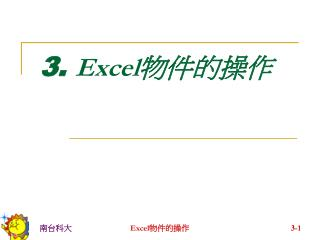DownloadDownload Presentation3. Excel 物件的操作

# 3. Excel 物件的操作

Télécharger la présentation## 3. Excel 物件的操作

- - - - - - - - - - - - - - - - - - - - - - - - - - - E N D - - - - - - - - - - - - - - - - - - - - - - - - - - -
##### Presentation Transcript

1. 3. Excel物件的操作 Excel物件的操作

2. 1. 物件變數 • Excel VBA = VB + Excel物件 • 常見Excel物件變數的宣告： Dim wb As Workbook ‘活頁簿 Dim s1 As WorkSheet ‘工作表 Dim ra As Range ‘範圍 Dim f1 As UserForm ‘表單 Dim sh As Shape ‘圖形 Dim c1 As Chart ‘圖表 Dim pt As PivotTable ‘樞紐分析表 • 加s的代表集合物件(複數)，如Worksheets Excel物件的操作

3. 屬性 方法 1.1 物件的屬性與方法 • 物件的組成包括： • (靜態)屬性(attributes) ：紀錄物件的各種性質。如，範圍的底色、字型、行數、列數等。(有些屬性是唯讀) • (動態)方法(methods)：物件的相關操作。如，範圍的copy、delete、select、activate等。 Excel物件的操作

4. 1.3 物件變數的語法 • 以 Set設定物件變數的值。 • 以dot指定物件的屬性、方法。 Sub 複製() Dim r As Range ‘宣告 r 為範圍物件變數 Set r = Range("A1:B1") '指定r的值為A1:B1 r.Copy'執行r的Copy方法 Set r = Range("A2:B2") '更改r的值為A2:B2 r.PasteSpecial‘執行r的選擇性貼上 r.Interior.Color = RGB(0, 255, 0) ‘儲存格背景設為綠色 Set r = Nothing‘清除r的內容 End Sub Excel物件的操作

5. 2. 活頁簿 Dim wb As Workbook Dim wb2 As Workbook Dim wb3 As Workbook ‘作用中活頁簿 Set wb = ActiveWorkbook ‘開新活頁簿 Set wb2 = Workbooks.Add ‘開啟舊檔(須包含完整路徑及檔名) Set wb3 = Workbooks.Open("D:\台糖\Excel\Excel 2003 VBA進階訓練班\EX1.xls") Excel物件的操作

6. ‘切換活頁簿 wb.Activate ‘切換活頁簿 wb2.CloseSaveChanges := False ‘False表不儲存關閉 ‘True表儲存後關閉 ‘省略則會出現詢問對話方塊 ‘另存新檔 wb.SaveAs ("D:\台糖\Excel\Excel 2003 VBA進階訓練班\qqq.xls") Excel物件的操作

7. ‘儲存檔案 wb3.Save ‘關閉所有活頁簿 Workbooks.Close Excel物件的操作

8. 3. 視窗 • 一個活頁簿會對應一個視窗 • 在VBA中，指定視窗的方式： Windows(3) Windows(“EX3”) • 視窗編號會因活頁簿的開啟、關閉而改變 • 活頁簿的Activate及關閉，也可藉由視窗來執行： Windows(3).Activate ‘切換視窗 Windows(“EX3”).Close ‘關閉視窗 Excel物件的操作

9. 調整視窗大小： ‘視窗最大化 Windows(2).WindowState = xlMaximized ‘視窗最大化 Windows(“EX1”).WindowState = xlMinimizes ‘視窗還原 Windows(“EX1”).WindowState = xlNormal Excel物件的操作

10. 4. 工作表 • 以名稱指定工作表： • 工作表名稱(字串)： Dim s2 As Worksheet Set s2 = Sheets("工作表名稱") Set s2 = Worksheets(“Sheet3”) • 工作表物件名稱(工作表)： Dim s2 As Worksheet ‘下行OK ! Set s2 = 工作表物件名稱 ‘!!! 下2行錯誤 Set s2 = Sheets("工作表物件名稱") Set s2 = Worksheets("工作表物件名稱") Excel物件的操作

11. 以索引指定工作表： • 工作表索引：工作表在活頁簿中，由左至右的順序；與專案總管中的編號不同。 • 工作表索引會因工作表的移動、新增、刪除而改變。專案總管中的編號則不變。 • Worksheets：只限一般工作表(Sheet1, Sheet2,...)，不含圖表工作表(Chart1, Chart2, …) • Sheets：包含一般工作表(Sheet1, Sheet2,...)及圖表工作表(Chart1, Chart2,...) Excel物件的操作

12. Dim s1 As Worksheet, s2 As Worksheet Dim c1 As Chart Set s1 = Sheets(3) ’與Set s1 = Worksheets(2) ’及Set s1 = Sheets(“工作表名稱”)相同 Set s2 = Worksheets(3) ‘與Set s2 = Sheets(4) ‘及Set s2 = Worksheets(“Sheet3”)相同 Set c1 = Sheets(1) ‘圖表工作表 Chart1 Set c1 = Worksheets(1) ‘!!!!! NG Set s1 = Sheets(1) ‘!!!!! NG Msgbox s2.Index ‘工作表索引 Msgbox s2.Name ‘工作表名稱 Excel物件的操作

13. 選取與啟動：工作表一經選取(select)，即被啟動(activate)而成為作用中工作表(ActiveSheet)。所以工作表的select和activate是一樣的。選取與啟動：工作表一經選取(select)，即被啟動(activate)而成為作用中工作表(ActiveSheet)。所以工作表的select和activate是一樣的。 Worksheets(“工作表名稱”).Select Sheets(4).Activate • 多工作表的選取：只能用select，不能用activate Worksheets(Array(2, 3)).Select Sheets(Array(2, 3)).Select Excel物件的操作

14. 隱藏/顯示工作表： Sheets(1).Visible = True ‘顯示 Sheets(1).Visible = False ‘隱藏，但可手動取消 Sheets(1).Visible = xlVeryHidden ‘隱藏且無法取消 或 Worksheets(1).Visible = True Worksheets(1).Visible = False Worksheets(1).Visible = xlVeryHidden Excel物件的操作

15. 新增： Sheets.Add • 刪除： Worksheets(1).Delete • 搬移： Sheets(“工作表名稱”).Move before:=Sheets(2) • 複製： Sheets(“工作表名稱”).Copy after:=Worksheets(3) Excel物件的操作

16. 5. 範圍 • 範圍的操作，在Excel VBA中相當重要，設定的方式有： • Range(“範圍”) Range(“A1”) ‘單一儲存格A1 Range(“B3:D5”) ‘連續範圍 Range(“B3:D5, A6:C9”) ‘不連續的範圍 Range(“B:B”) ‘B行 Range(“2:7”) ‘2~7列 • Range(儲存格A，儲存格B) Range(“B3”, “D5”) ‘連續範圍 Range(ActiveCell, “A1”) Excel物件的操作

17. 5.1 範圍中的不連續區塊(範圍) Dim r1 As Range, r2 As Range '------------- Areas Set r1 = Range("B3:D5, A6:C9") Set r2 = r1.Areas(2)'Areas(1 To N) MsgBox r2.Address‘A6:C9 For Each r2 In r1.Areas MsgBox r2.Address Next r2 Excel物件的操作

18. 5.2 範圍的聯集與交集 Dim r1 As Range, r2 As Range ‘聯集 Set r1 = Union(Range("a1:b3"), Range("c5:d6")) ‘交集 Set r2 = Intersect(Range("a1:b3"),Range("b2:d5")) Excel物件的操作

19. 5.3 範圍的邊界 • Ctrl + Shift + 8：包含目前位置的連續範圍 Dim r1 As Range Set r1 = ActiveCell.CurrentRegion • Ctrl + 方向鍵：以目前位置為起點，連續範圍的邊界 ActiveCell.End(xlUp).Select‘連續的上邊界 ActiveCell.End(xlDown).Select‘連續的下邊界 ActiveCell.End(xlToLeft).Select‘連續的左邊界 ActiveCell.End(xlToRight).Select‘連續的右邊界 Excel物件的操作

20. Range的邊界： Dim r1 As Range Set r1 = Range(“C4:F11”) MsgBox r1.Row & “,” & r1.Column‘r1左上角3,4 ‘r1的列數,行數 MsgBox r1.Rows.Count & “," & r1.Columns.Count Excel物件的操作

21. 5.4 欄列的選取 • Rows ‘所有列 • Rows(“5:5”) ‘第5列 • Rows(5) • Rows(“3:6”) ‘第3~6列 • Columns ‘所有行 • Columns(“D:D”) ‘第4行 • Columns(4) • Columns(“D”) • Columns(“E:H”) ‘第5~8行 • Range(“C4:F11”).Rows(“2:3”) ‘C5:F6 • Range(“C4:F11”).Columns(“C:C”) ‘E4:E11 Excel物件的操作

22. 6. 儲存格 • Range(“B3”) • Cells(3, 2) ‘Cells(列，行) • Cells(3, “B”) • Cells(10) ‘第10個儲存格(row major) • Cells ‘所有儲存格 • [B10] ‘B10，也能設定範圍[B10:C12] Excel物件的操作

23. Range可以設定儲存格及範圍，缺點是不適合搭配For-NextRange可以設定儲存格及範圍，缺點是不適合搭配For-Next • Cells適合搭配For-Next操作，缺點是無法指定範圍 • 以Cells設定Range範圍： Range(“C4:F11”) Range(Cells(4,3), Cells(11,6)) ‘結合兩者優點 Excel物件的操作

24. 7. 相對參照 • Range/Cells.Range/Cells Dim r1 As Range Range("c4:f11").Range("b2").Select 'D5 MsgBox ActiveCell.Address Range("g12").Select Set r1 = ActiveCell.Range(“a1:b2”) 'G12:H13 MsgBox r1.Address Range("c4:f11").Cells(3, 2).Select 'D6 MsgBox ActiveCell.Address Excel物件的操作

25. Offset(列位移，行位移)： [b10].Offset(2, 3).Select 'E12 Cells(10, 2).Offset(-1,1) ‘C9 Range(“C4”).Select ActiveCell.Offset(1, -1) ‘B5 Excel物件的操作